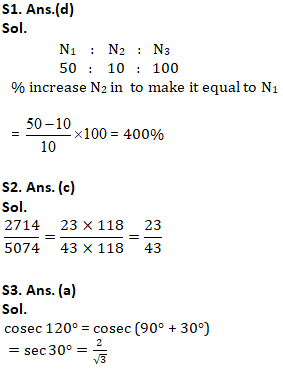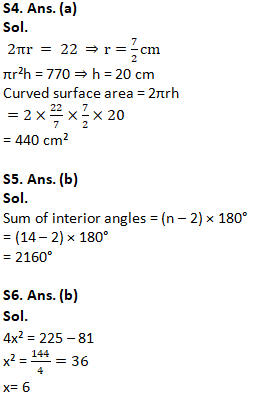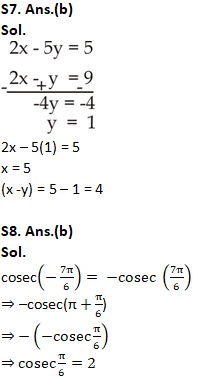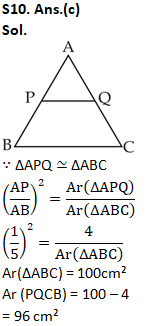Latest SSC jobs   »   Mathematics Quiz For RRB NTPC :...

# Mathematics Quiz For RRB NTPC : 18th January 2020 for Percentage and Number System

Q1. Two numbers are 50% and 90% lesser than a third number. By how much percent is the second number to be enhanced to make it equal to the first number?
(a) 80 percent
(b) 40 percent
(c) 44.44 percent
(d) 400 percent

Q2. Reduce 2714/5074 to lowest terms.
(a) 17/23
(b) 29/43
(c) 23/43
(d) 31/37

Q3. What is the value of cosec 120°
(a) 2/√3
(b) 2
(c) -2/√3
(d) -2

Q4. Volume of a cylinder is 770 cubic cm. If circumference of its base is 22 cm, what will be the curved surface area of the cylinder? (Take π = 22/7)
(a) 440 sq cms
(b) 880 sq cms
(c) 220 sq cms
(d) 660 sq cms

Q5. What will be the sum of the measures all the interior angles of a polygon having 14 sides?
(a) 2520°
(b) 2160°
(c) 2880°
(d) 3240°

Q6. If 4×2 = 152 – 92, then value of x is
(a) 9
(b) 6
(c) 3
(d) 12

Q7. If 2x – 5y = 5 and 2x – y = 9, then x – y is ______.
(a) 2
(b) 4
(c) 6
(d) 3

Q8. What is the value of cosec( -7π/6)?
(a) -2
(b) 2
(c) 2/√3
(d) -2/√3

Q9. The diagonals do not form at least two congruent triangles in a _____.
(a) Parallelogram
(b) Rhombus
(c) Trapezium
(d) Kite

Q10. Points P and Q lie on side AB and AC of triangle ABC respectively such that segment PQ is parallel to side BC. If the ratio of AP:PB is 1:4 and area of Δ APQ is 4 sq cm, what is the area of trapezium PQCB?
(a) 60 sq cm
(b) 16 sq cm
(c) 96 sq cm
(d) 21 sq cm

Solutions:S9. Ans.(c)
Sol.
Except trapezium, all other figures have at least two congruent triangles.##### RRB NTPC 2019 | RRB JE Classes Day 1 | Review of Previous Year Quant Papers for RRB | Sumit Sir

Important Links for RRB NTPC Recruitment 2019
RRB NTPC Previous year Cut Off | 1st & 2nd Stage Examination
RRB NTPC Recruitment 2019: Check FAQs
RRB NTPC Exam Pattern 2019 – Check Here
RRB NTPC Previous Year Exam Analysis
RRB NTPC Exam Syllabus#### Congratulations!General Awareness & Science Capsule PDF